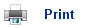# Dynamic Equilibrium and Equilibrium Constants

• If a reaction is left to continue in a sealed system, then eventually the reaction will reach a dynamic equilibrium.
• This is where the concentration of the reactants and products remains constant.
• Thus, at a dynamic equilibrium, the forward reaction will occur at the same rate as the backward reaction.
• In “minerals to elements” the position of equilibrium was discussed. That is what effect changing the temperature, concentration, or pressure had on the reaction.
• The effects could be predicted using La Chatelier’s Principle which states that if a system is at equilibrium, and a change is made in one of the conditions, then the system responds to counteract the change as much as possible.
• However, as chemists, it is not good enough just to be able to say that the position of equilibrium is to the left or the right; there needs to be a measure of how far left or right the position of equilibrium is; this is where equilibrium constants are of use.

## Equilibrium Constants

• For a thorough understanding of chemical equilibrium, we need to be able to look at quantities of the substances involved; thus in a solution concentrations are used.
• The following reaction is at a dynamic equilibrium:

H2(g) + I2(g)2HI(g)

• The table below shows the concentrations of the different reaction mixtures measured (determined experimentally) when the reaction reached equilibrium (equilibrium concentrations):• It was determined that all three reactions would fit into the expression: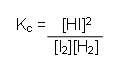• Meaning that the same Kc value is constant for all three reactions. The Kc is the equilibrium constant for this reaction: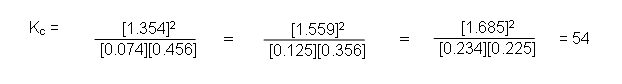• In general, for the reaction:

aA + bBcC + dD

• The equilibrium constant (Kc) will equal: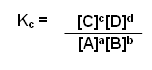• So, to write an equilibrium constant for a reaction, you must:
1. Write a balanced equation for the reaction.
2. Take the concentrations of the products and raise them to the power of the number which appears in front of it in the balanced equation.
3. Divide them by the concentration of reactants raised to the power of the number in front of them in the balanced equation.

For example:
Write down an equation to show the equilibrium constant for the formation of ammonia from nitrogen and hydrogen:

1. Write a balanced equation:

N2(g) + 3H2(g)2NH3(g)

2. Raise the concentration of the products to the power of the number in front of them:

[NH3]2

3. Divide them by the concentration of the reactants raised to the power of the number in front of them: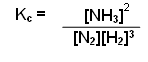• The equilibrium constant is only applicable when the reaction is in a closed system, and the temperature is kept constant; i.e. the equilibrium constant will change if the temperature is altered.

## The units of equilibrium constants

• The units of Kc vary; it depends on the expression for Kc for the particular reaction being studied.

Example 1:

H2(g) + Br2(g)2HBr(g)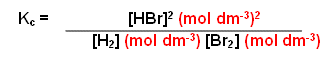• For this reaction, there are no units, because the units on the bottom cancel those on the top.

Example 2:

N2(g)+ 3H2(g)NH3(g)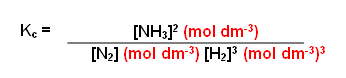• For this reaction, the overall units are (mol dm-3)-2, or simplified mol-2 dm-6.

## Changing the reaction

• It does not matter whether the reaction started with the reactants and no products, or the products and no reactants; the concentrations of the reactants and products will still be the same at equilibrium.
• However, reversible reactions can be written in two ways, for example:

N2(g) + 3H2(g)2NH3(g)

2NH3(g)N2(g) + 3H2(g)

• This will have an effect on the equilibrium constant: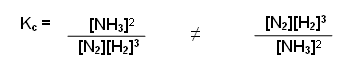• As you can see, the KC expressions are the reciprocals of each other, therefore 1/Kc for the first reaction will give the Kc for the seconds reaction (the units are also reciprocals, i.e. for the first it will be mol-2 dm6 and for the second it will be mol2 dm-6).

## Example Question:

For the reaction

2H2(g) + S2(g)2H2S(g)

The Kc was found to be 9.4 x 105 mol-1 dm3 at 1020 K. Equilibrium concentrations were measured as:

[H2(g)] = 0.234 mol dm-3
[H2S(g)] = 0.442 mol dm-3

What is the equilibrium concentration of S2(g) in the above mixture?
1. First of all, write out the rate equation: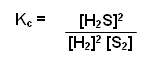2. Substitute in the known values: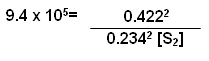3. Rearrange and solve algebraically: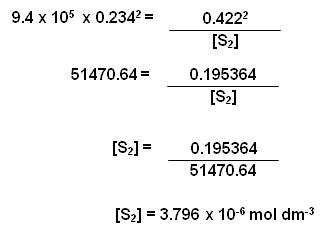## Useful books for revision

Revise A2 Chemistry for Salters (OCR A Level Chemistry B)Salters (OCR) Revise A2 ChemistryHome